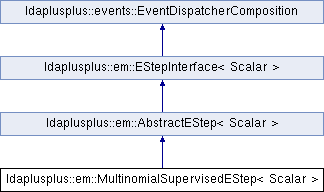LDA++
ldaplusplus::em::MultinomialSupervisedEStep< Scalar > Class Template Reference
Inheritance diagram for ldaplusplus::em::MultinomialSupervisedEStep< Scalar >:## Public Member Functions

MultinomialSupervisedEStep (size_t e_step_iterations=10, Scalar e_step_tolerance=1e-2, Scalar mu=2, Scalar eta_weight=1, Scalar compute_likelihood=1.0, int random_state=0)

std::shared_ptr< parameters::Parametersdoc_e_step (const std::shared_ptr< corpus::Document > doc, const std::shared_ptr< parameters::Parameters > parameters) overridePublic Member Functions inherited from ldaplusplus::em::AbstractEStep< Scalar >
AbstractEStep (int random_state)

virtual void e_step () overridePublic Member Functions inherited from ldaplusplus::events::EventDispatcherComposition
std::shared_ptr< EventDispatcherInterfaceget_event_dispatcher ()

void set_event_dispatcher (std::shared_ptr< EventDispatcherInterface > dispatcher)Protected Member Functions inherited from ldaplusplus::em::AbstractEStep< Scalar >
bool converged (const Eigen::Matrix< Scalar, Eigen::Dynamic, 1 > &gamma_old, const Eigen::Matrix< Scalar, Eigen::Dynamic, 1 > &gamma, Scalar tolerance)

PRNGget_prng ()

## Constructor & Destructor Documentation

template<typename Scalar >
 ldaplusplus::em::MultinomialSupervisedEStep< Scalar >::MultinomialSupervisedEStep ( size_t e_step_iterations = 10, Scalar e_step_tolerance = 1e-2, Scalar mu = 2, Scalar eta_weight = 1, Scalar compute_likelihood = 1.0, int random_state = 0 )
Parameters
 e_step_iterations The max number of times to alternate between maximizing for $$\gamma$$ and for $$\phi$$. e_step_tolerance The minimum relative change in the variational parameter $$\gamma$$. mu The uniform Dirichlet prior of $$\eta$$, practically is a smoothing parameter during the maximization of $$\eta$$. eta_weight A weighting parameter that either increases or decreases the influence of the supervised part. compute_likelihood The percentage of documents to compute likelihood for (1.0 means compute for every document) random_state An initial seed value for any random numbers needed

## Member Function Documentation

template<typename Scalar >
 std::shared_ptr< parameters::Parameters > ldaplusplus::em::MultinomialSupervisedEStep< Scalar >::doc_e_step ( const std::shared_ptr< corpus::Document > doc, const std::shared_ptr< parameters::Parameters > parameters )
overridevirtual

Maximize the ELBO w.r.t. $$\phi$$ and $$\gamma$$.

The following steps are the mathematics that are implemented where $$\beta$$ are the over words topics distributions, $$\alpha$$ is the Dirichlet prior, $$\eta$$ are the logistic regression parameters, $$i$$ is the topic subscript, $$n$$ is the word subscript, $$\hat{y}$$ is the class subscript, $$y$$ is the document's class, $$w_n$$ is n-th word vocabulary index, $$m$$ is a weighting parameter used to adjust the influence of the supervised part and finally $$\Psi(\cdot)$$ is the first derivative of the $$\log \Gamma$$ function.

1. Repeat until convergence of $$\gamma$$.
2. Compute $$\phi_{ni} \propto \beta_{iw_n}\eta_{yi}^m\exp\left( \Psi(\gamma_i)\right)$$
3. Compute $$\gamma_i = \alpha_i + \sum_n^N \phi_{ni}$$
Parameters
 doc A single document. parameters An instance of class Parameters, which contains all necessary model parameters for e-step's implementation.
Returns
The variational parameters for the current model, after e-step is completed.

Implements ldaplusplus::em::EStepInterface< Scalar >.

The documentation for this class was generated from the following files: